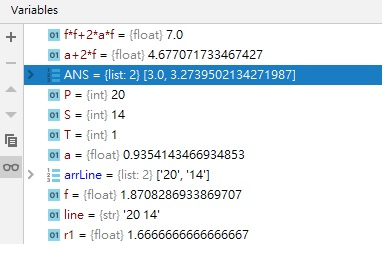# J7 - The Best Box guidance

http://www.codechef.com/problems/J7 codechef J7 - “the best box” guidance:

``````- to get optimal maximum size: (special thanks to falcon - Sahin Mammadov) suppose we have 2 functions "a" & "b". function "f(x)=a*b" and constant "c=a+b". b=c-a, from that f(x)=a*b, f(x)=a*(c-a)=a*c-a**2. to get that functions maximum, we get their derivatives(look at images below).

maximum function

max and min function

and after derivative f '(x)=c-2*a=0, a=c/2, b=c-a=c-(c/2)=c/2. so from the equation there we understand that to get optimal maximum "a" and "b" needs to be equal.

- calculation of volume of rectangle, given surface area and perimeter: suppose all sides are not equal: P(perimeter)=4*(a+b+c), S(surface area)=2*a*b+2*a*c+2*b*c and V(volume)=a*b*c. but on the above calculation i've shown that at least two sides needs to be equal to get optimal maximum. so,  a=b, (but here i will put "b" intsead of "c") P(perimeter)=4*(a+a+b), S(surface area)=2*a*a+2*a*b+2*a*b and V(volume)=a*a*b.

next step, P/4=2*a+b, S=2*a**2+4*a*b take square of P/4 side: (P/4)**2=(2*a+b)**2=4*a**2+4*a*b+b**2=2*a**2+4*a*b+2*a**2+b**2=S+2*a**2+b**2; (P/4)**2=S+2*a**2+b**2; next step, P/4=2*a+b, from there P/4-2*a=b. and then put P/4-2*a instead of b at the equation above: (P/4)**2=S+2*a**2+(P/4-2*a)**2. from there we get: 6*a**2-P*a+S=0; and now apply discrimination formula(shown below): a1,a2=(P+-sqrt(P**2-24*S))/12;

discrimination

and after all, we apply a1,a2 to "b" and "V"(volume): V=a**2*b, P/4-2*a=b; V=((P+-sqrt(P**2-24*S))/12)**2*b =
=((P+-sqrt(P**2-24*S))/12)**2 * P/4-2*a
=((P+-sqrt(P**2-24*S))/12)**2 * P/4-2*((P+-sqrt(P**2-24*S))/12);

but i actually printed out, ((P-sqrt(P**2-24*S))/12) instead of ((P+sqrt(P**2-24*S))/12), and got AC, dont know why. (it would be nice if you rectify me)

and also to print out 2 digits after ","(comma) use include <iomanip> and cout<<fixed<<setprecision(2)<<etc.

thanks. any cooperation will be appreciated

NOTE: "x**2" means pow(x, 2), x power of 2
``````4 Likes

V=aab;
where b=p-2a; where p=wire length/4;
Hence,V=a
ap-2aaa
this is dec fn in a (if p>3)
hence for lower value of a ,V is more
Therefore
((P-sqrt(P**2-24*S))/12)
gave you correct ans

For 6a**2-Pa+S=0 to yield maximum value of a , its double derivative should be less than 0 i.e 12a-P<0
or a<P/12. — Equation 1 Therefore maximum value of a can be P/12.
Now, (P±sqrt(P**2-24
S))/12 can be written as (P/12)±sqrt(P**2-24S)/12 .Therefore only “-” sign will result in correct value of a.(From equation 1).
Thus it follows that ((P-sqrt(P
2-24S))/12) gave you correct ans

It will be a cuboid instead of rectangle :)!

1 Like

i spent quite a lot of time on this problem —(the best box, aka codeId:J7), in recent a few days.

(Honestly, i don’t understand the correctness of the posts of garakchys’; so i ignore them)

After my study, I have to propose some wieldness in the given official test cases:
on official page(https://www.codechef.com/problems/J7), in first test case,
they said (3,1,1) forms largest box conforming ( a+b+c<=5 and ab+bc+ac<=7)If we apply a=0.935414, b=c=1.870829=f
a+2f=4.67
f^2 + 2af= 7.0
the volume of this new tuple (0.935414,1.870829, 1.870829)
seems to be bigger than 3 ( 3.27395=a*(f^2) > 3)

am I wrong?
shall i put official comment on that J7 page?

Sorry, I omitted one important statement—“we should use all the wires and papers…”